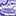# QlikView App Dev

Discussion Board for collaboration related to QlikView App Development.

Announcements
Join us at the Cloud Data and Analytics Tour! REGISTER TODAY
cancel
Showing results for
Search instead for
Did you mean:Not applicable

## Variable records in a field

Hi All,

I have a table that has a field call 'ForecastVersion' this table can contain 3 records, the 'Current' froecast and the previous two forecasts, for example, '1H13' and '2H13'. The current forecast will always be called 'Current' bur the names for the previous two forecasts will change every 6 months. For example, shortly we will roll to a new forecast so the previous two forecasts will become '2H13' and '1H14'

In my Qlikview app, I have a lot of tables and charts that compare the current forecast with the prev two. Given that I don't want to hardwire the forecast names into the expressions etc can you suggest a way that I can make my code independent of the forecast version names? Can I assign \$expansion variables to the data during import? (from an excel flat file)

Thanks for the help

John

6 RepliesMVP & Luminary

I think the easiest way would be to use fixed columns/fields for the previous forecasts, like previous1 and previous2 and only the labels are dynamically per additionally mapping-fields or variables or expressions, see this as example:

set vprevious1 = '2H13'; //script or gui

label: \$(vprevious1)

exp: sum(previous1)

- MarcusNot applicable
Author

Hi Marcus,

Thanks for the reply. What I have actually done is set variables

LETvCurrent_Forecast = 'Current'; //the most recent forecast, typically Current
LET vLast_Forecast = 'POR 2H13';
LET vPreviousToLast_Forecast = 'POR 1H13'

Then I use the variable in my expression. However, I hit a problem that maybe you can help with. When I use the variables in an expression, the 'Current' one works fine:

Sum({\$<ForecastVersion={\$(vCurrent_Forecast)}>}Data)

But when I apply the same expression to vLastForecast I don't get any data back:

Sum({\$<ForecastVersion={\$(vPrevious_Forecast'}>}Data)

I think this is because I have a space between the 'POR' and '2H13'. I tried aplying [ ] but can't get it to work. Any ideas?

I also tried a different route and greated a ForecastVersion table usine a LOAD DISTINCT to generate a table with the 3 forecast names in it. I'm thinking I can use this in my experssions. How can I incorporate this into an expression such that I can say something like:

Sum({\$<ForecastVersion={table Row 1}>}Data)

Sum({\$<ForecastVersion={table Row 2}>}Data)

Sum({\$<ForecastVersion={table Row 3}>}Data)

All help appreciated...

Thanks

JohnMVP

Is it vPreviousToLast_Forecast or vPrevious_Forecast?

Try enclosing it in single quotes:

Sum({\$<ForecastVersion={'\$(vPrevious_Forecast)'}>}Data)

talk is cheap, supply exceeds demandNot applicable
Author

Hi Gysbert,

Thanks for the hintI was addressing the incorrect variable name. All sorted nowCan you help with his question?

I also tried a different route and greated a ForecastVersion table usine a LOAD DISTINCT to generate a table with the 3 forecast names in it. I'm thinking I can use this in my experssions. How can I incorporate this into an expression such that I can say something like:

Sum({\$<ForecastVersion={table Row 1}>}Data)

Sum({\$<ForecastVersion={table Row 2}>}Data)

Sum({\$<ForecastVersion={table Row 3}>}Data)

JohnMVP

Perhaps something like this:

Sum({\$<ForecastVersion={"\$(=FieldValue('MyForecastField',1))"}>}Data)

Sum({\$<ForecastVersion={"\$(=FieldValue('MyForecastField',2))"}>}Data)

Sum({\$<ForecastVersion={"\$(=FieldValue('MyForecastField',3))"}>}Data)

talk is cheap, supply exceeds demandPartner

try this

Sum({\$<ForecastVersion={"\$(vPrevious_Forecast)"}>}Data)